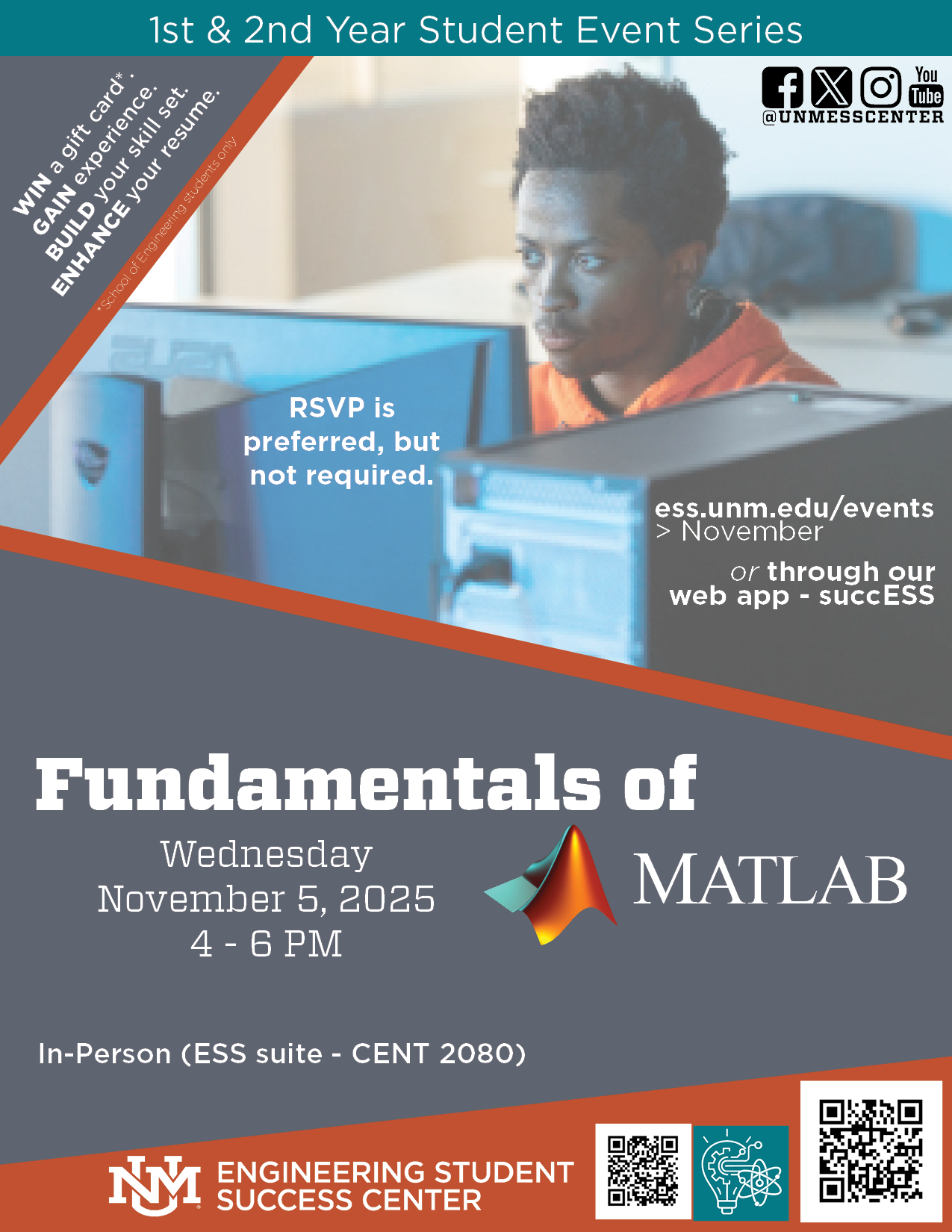# Fundamentals of MATLABMATLAB is a high-performance language utilized for technical and scientific computations. With a user-friendly interface, one can apply such a tool to their disposal to accomplish any sort of data analysis, calculation, and algorithm development. Whether it comes to an arduous or strenuous mathematical problem to the statistical extrapolation of graphical information, MATLAB will benefit the user in terms of efficiency and trumps results over efforts anytime.

In this 2.5 hour introductory hands-on workshop, one will learn:

• The importance of MATLAB
• Comprehend programming etiquette
• Gain the basics to be independent and create more complex programs

Presented by: Michael Tanguay - Senior - Nuclear Engineering, Mathematics & Computer Science